# 连续函数……你可以笔不离纸地把线画下来……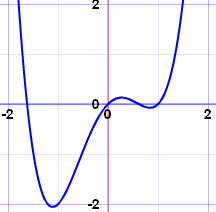## 例子：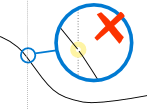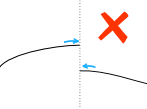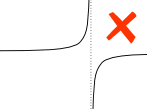非连续 非连续 非连续 （缺口） （间隙） （垂直渐近线）

（用滑标可以放大/缩小、拖曳可以移位、点击可以置于中部。）

## 定义域

 函数有个定义域。 定义域最简单的意思就是所有可以代入函数的值。### 例子：1/(x-1)

1/(1-1) = 1/0 = 未定义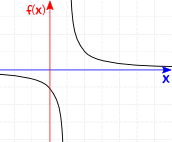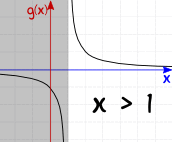f(x) = 1/(x-1) 对于全部 整数 g(x) = 1/(x-1) 对于 x>1 非连续 连续

g(x) 并包括 x=1，所以它是连续的。

## 更正式的解释！

f(c) 是 已定义的，并且：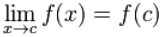"当 x 趋近 c 时，f(x) 的极限等于 f(c)"

"当 x 越来越趋近 c 时
f(x) 便越来越趋近 f(c)"

 x （从左边）趋近 c f(x) 便趋近 f(c)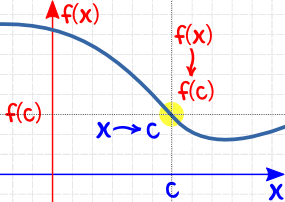并且 x （从右边）趋近 c f(x) 便趋近 f(c)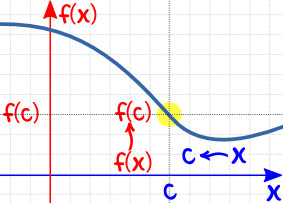## 怎样使用：

• f(x) 是已定义的
• x 的极限等于 f(x)

### 例子：f(x) = (x2-1)/(x-1) 对于所有实数(x2-1)/(x-1) = (12-1)/(1-1) = 0/0

### 例子：这个 分段函数 呢？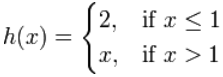图像这样：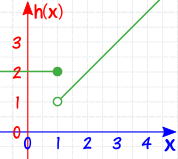• "2" （从左边），
• "1" （从右边）

### 例子：这个分段函数――绝对值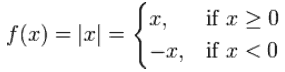图象这样：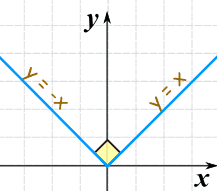（但函数不是 可微分的。）